# ConvR: Adaptive Convolution for Multi-Relational Learning

## Basic Idea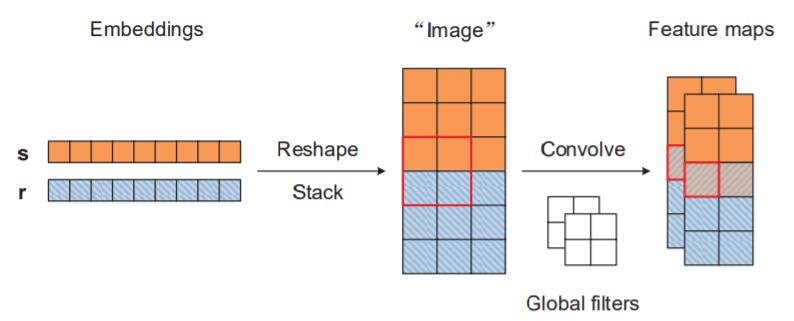## ConvR

ConvR其实非常简单, 它仍然没有脱离ConvE的框架. 它仅仅只是将卷积核直接拿掉了, “关系就是卷积核“.

ConvR的精髓在于, 适应性的将Relation Embedding构建成卷积核, 与Entity Embedding交互: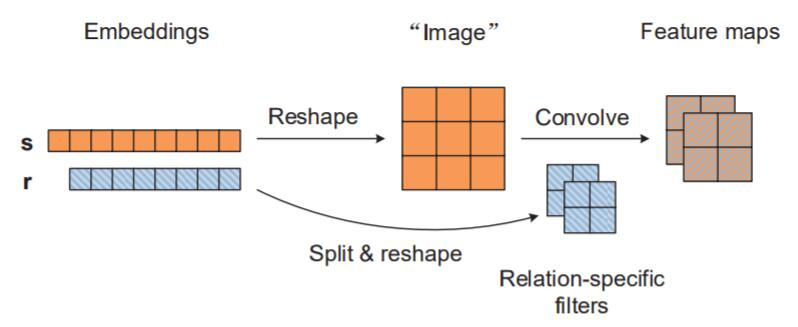#### The ConvR Model

$$c_{m, n}^{(\ell)}=f\left(\sum_{i, j} s_{m+i-1, n+j-1} \times r_{i, j}^{(\ell)}\right)$$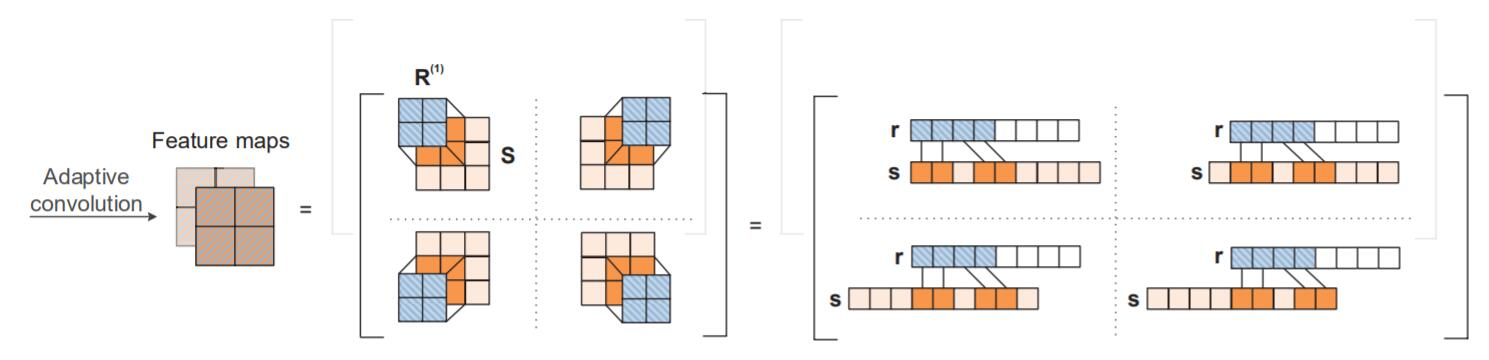$$\psi(s, r, o)=f(\mathbf{W} \mathbf{c}+\mathbf{b})^{\top} \mathbf{o}$$

### Parameter Learning

$$p_{o}^{s, r}=\sigma(\psi(s, r, o))$$

$$\mathcal{L}(s, r)=- \frac{1}{|\mathcal{E}|} \sum_{o \in \mathcal{E}} y_{o}^{s, r} \log \left(p_{o}^{s, r}\right)+ \left(1-y_{o}^{s, r}\right) \log \left(1-p_{o}^{s, r}\right)$$

1. 交互次数比ConvE变多了, 每次卷积都是Entity Embedding和Relation Embedding的交互.

2. 参数比ConvE少了, 因为”关系就是卷积核“.

ConvE:$\mathcal{O}\left(d|\mathcal{E}|+d|\mathcal{R}|+c h w+c d\left(2 d^{h}-h+1\right)\left(d^{w}- w + 1\right)\right)$

ConvR: $\mathcal{O}\left(d_{e}|\mathcal{E}|+d_{r}|\mathcal{R}|+c d_{e}\left(d_{e}^{h}-h+1\right)\left(d_{e}^{w}-w+1\right)\right)$

ConvR少掉的部分$chw$ 被集成进了Relation Embedding中.

## Experiments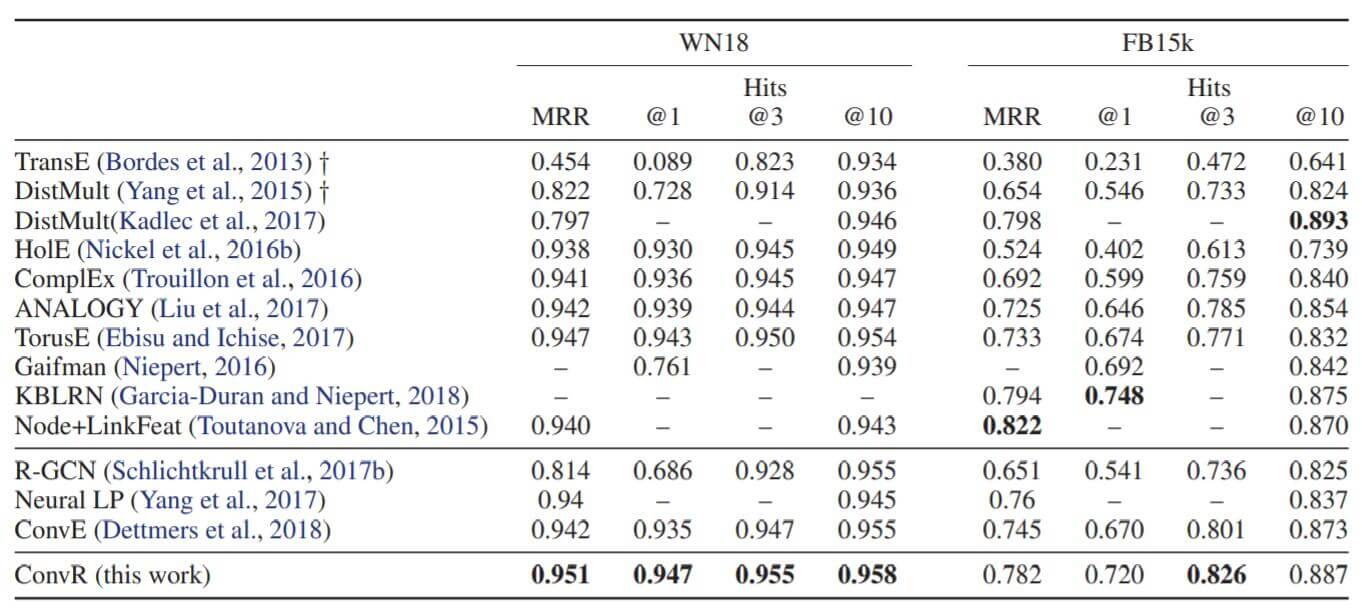WN18和FB15K这两个数据集是存在大量逆关系的, 结果可能是过拟合了的.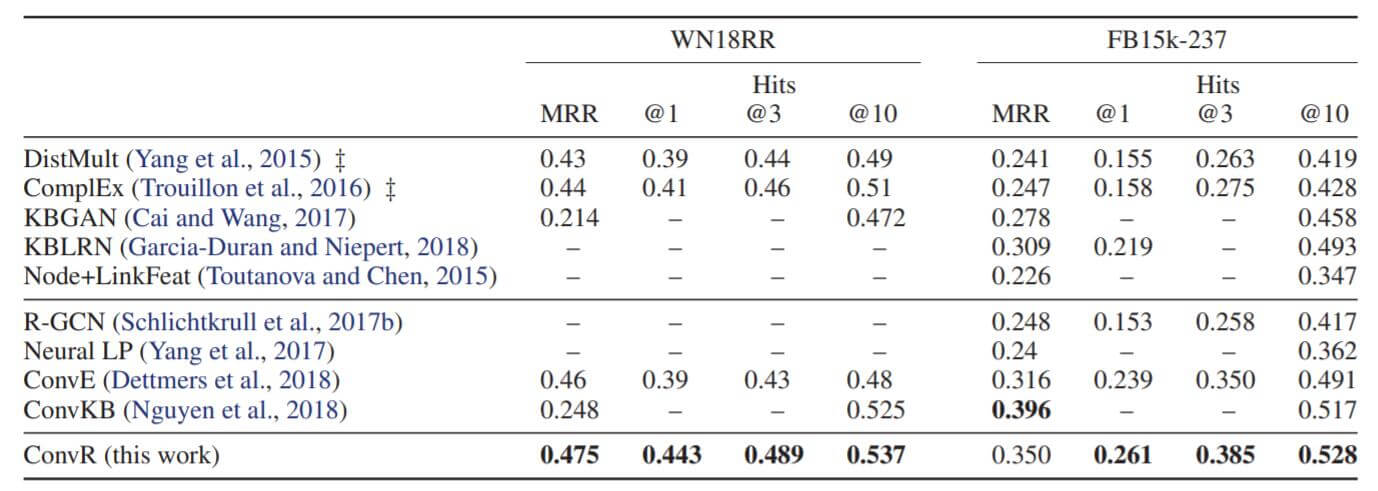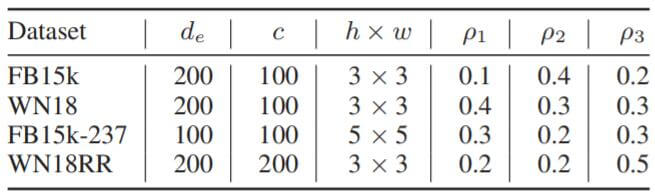### Parameter Efficiency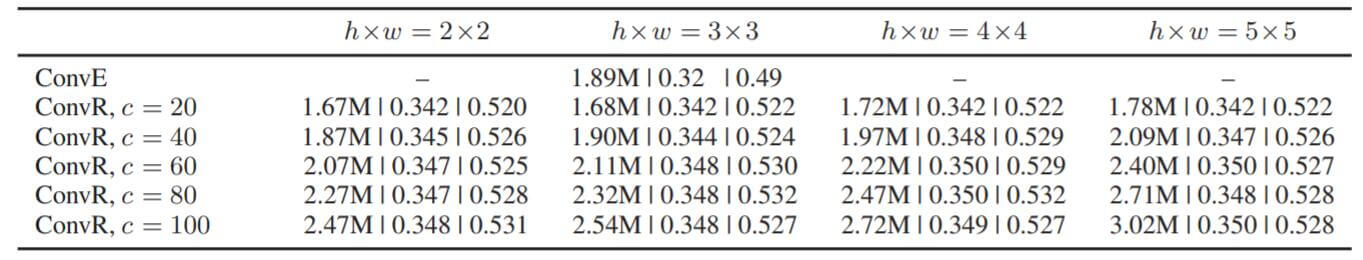## Summary

• 虽然提升了关系和实体之间的交互次数, 但受制于直接拿关系Embedding作为卷积核的方式, ConvR无法像其他卷积类方法把模型做得更. 一般来讲, 卷积层数越深, 所抽取到的特征也就越高阶, 也就意味着更复杂的实体与关系交互, 但在ConvR中只能做一层卷积.
• 因为卷积的特殊机制, $d_e$ 和$d_r$ 大概率不相同, 可能会对下游任务使用产生一定影响(许多场景要求$d_e$ 和$d_r$ 是相同维度的, 所以还需要额外维度压缩). 作者所给出的示例$d_r$ 都比较大, 如果强行调整至一致可能会导致性能衰减.

上一篇Pytorch实现: BERT

2021-03-12LightCAKE: A Lightweight Framework for Context-Aware Knowledge Graph Embedding
2021.03.09: 修正关于引入逆三元组的影响. 2021.04.18: 更新一篇更早的类似论文GAKE. LightCAKE: A Lightweight Framework for Context-Aware Knowledge
2021-03-08
目录